# Plotting and Interactivity## Plotting and Jupyter Notebooks

#### Unidata Python Workshop

One of the most common tasks we face as scientists is making plots. Visually assessing data is one of the best ways to explore it - who can look at a wall of tabular data and tell anything? In this lesson we'll show how to make some basic plots in notebooks and introduce interactive widgets.

Matplotlib has many more features than we could possibly talk about - this is just a taste of making a basic plot. Be sure to browse the matplotlib gallery for ideas, inspiration, and a sampler of what's possible.

In :
# Import matplotlib as use the inline magic so plots show up in the notebook
import matplotlib.pyplot as plt
%matplotlib inline

In :
# Make some "data"
x = [1, 2, 3, 4, 5, 6, 7, 8, 9, 10]
y = [2, 4, 8, 16, 32, 64, 128, 256, 512, 1024]


### Basic Line and Scatter Plots¶

In :
# Make a simple line plot
plt.plot(x, y)

Out:
[<matplotlib.lines.Line2D at 0x7f7b6ac2d210>]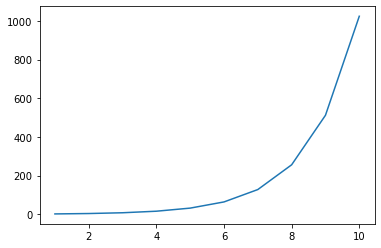In :
# Play with the line style
plt.plot(x, y, color='tab:red', linestyle='--')

Out:
[<matplotlib.lines.Line2D at 0x7f7b6ab31150>]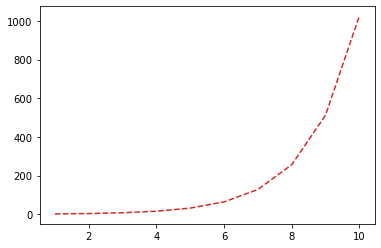In :
# Make a scatter plot
plt.plot(x, y, color='tab:orange', linestyle='None', marker='o')

Out:
[<matplotlib.lines.Line2D at 0x7f7b6aa9a850>]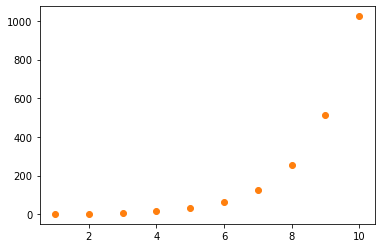### Adding Interactivity to Plots¶

In :
# Let's make some more complicated "data" using a sine wave with some
# noise superimposed. This gives us lots of things to manipulate - the
# amplitude, frequency, noise amplitude, and DC offset.
import numpy as np

x = np.linspace(0, 2*np.pi, 100)
y = 10 * np.sin(x) + np.random.random(100)*5 + 20

In :
# Have a look at the basic form of the data
plt.plot(x, y)
plt.xlabel('X Values')
plt.ylabel('Y Values')
plt.title('My Temperature Data')

Out:
Text(0.5, 1.0, 'My Temperature Data')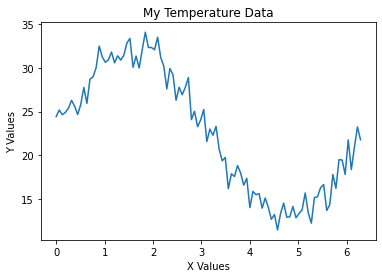In :
# Let's add some interactive widgets

from ipywidgets import interact

def plot_pseudotemperature(f, A, An, offset):
x = np.linspace(0, 2*np.pi, 100)
y = A * np.sin(f * x) + np.random.random(100) * An + offset

fig = plt.figure()
plt.plot(x, y)
plt.xlabel('X Values')
plt.ylabel('Y Values')
plt.title('My Temperature Data')
plt.show()

interact(plot_pseudotemperature,
f = (0, 10),
A = (1, 5),
An = (1, 10),
offset = (10, 40))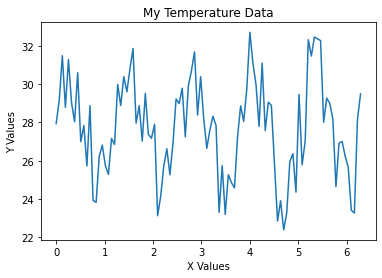Out:
<function __main__.plot_pseudotemperature(f, A, An, offset)>
In :
# We can specify the type of slider, range, and defaults as well

from ipywidgets import FloatSlider, IntSlider

def plot_pseudotemperature2(f, A, An, offset, title):
x = np.linspace(0, 2*np.pi, 100)
y = A * np.sin(f * x) + np.random.random(100) * An + offset

fig = plt.figure()
plt.plot(x, y)
plt.xlabel('X Values')
plt.ylabel('Y Values')
plt.title(title)
plt.show()

interact(plot_pseudotemperature2,
f = IntSlider(min=1, max=7, value=3),
A = FloatSlider(min=1, max=10, value=5),
An = IntSlider(min=1, max=10, value=1),
offset = FloatSlider(min=1, max=40, value=20),
title = 'My Improved Temperature Plot')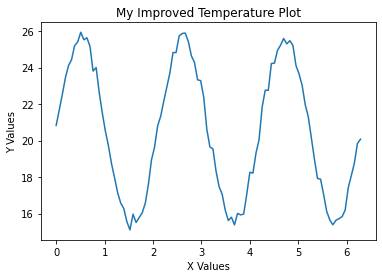Out:
<function __main__.plot_pseudotemperature2(f, A, An, offset, title)>